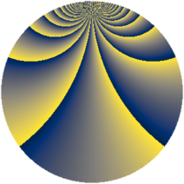# Properties

 Label 784.2.xLevel $784$ Weight $2$ Character orbit 784.x Rep. character $\chi_{784}(165,\cdot)$ Character field $\Q(\zeta_{12})$ Dimension $304$ Newform subspaces $16$ Sturm bound $224$ Trace bound $5$

# Related objects

## Defining parameters

 Level: $$N$$ $$=$$ $$784 = 2^{4} \cdot 7^{2}$$ Weight: $$k$$ $$=$$ $$2$$ Character orbit: $$[\chi]$$ $$=$$ 784.x (of order $$12$$ and degree $$4$$) Character conductor: $$\operatorname{cond}(\chi)$$ $$=$$ $$112$$ Character field: $$\Q(\zeta_{12})$$ Newform subspaces: $$16$$ Sturm bound: $$224$$ Trace bound: $$5$$ Distinguishing $$T_p$$: $$3$$, $$5$$

## Dimensions

The following table gives the dimensions of various subspaces of $$M_{2}(784, [\chi])$$.

Total New Old
Modular forms 480 336 144
Cusp forms 416 304 112
Eisenstein series 64 32 32

## Trace form

 $$304 q + 2 q^{2} + 2 q^{3} + 6 q^{4} + 2 q^{5} + 8 q^{6} - 4 q^{8} + O(q^{10})$$ $$304 q + 2 q^{2} + 2 q^{3} + 6 q^{4} + 2 q^{5} + 8 q^{6} - 4 q^{8} - 4 q^{10} + 10 q^{11} + 2 q^{12} + 8 q^{13} - 32 q^{15} - 18 q^{16} + 4 q^{17} - 44 q^{18} + 2 q^{19} - 8 q^{20} + 16 q^{22} - 18 q^{24} + 2 q^{26} + 20 q^{27} - 48 q^{29} - 34 q^{30} - 20 q^{31} - 8 q^{32} + 4 q^{33} + 32 q^{34} + 80 q^{36} + 18 q^{37} - 18 q^{38} - 50 q^{40} + 12 q^{44} + 28 q^{45} - 24 q^{46} + 44 q^{47} - 40 q^{48} - 76 q^{50} + 6 q^{51} - 4 q^{52} - 14 q^{53} - 82 q^{54} + 24 q^{58} + 18 q^{59} - 26 q^{60} + 2 q^{61} - 28 q^{62} - 132 q^{64} + 4 q^{65} - 2 q^{66} - 50 q^{67} - 24 q^{68} + 20 q^{69} + 62 q^{72} + 52 q^{74} + 24 q^{75} + 68 q^{76} - 204 q^{78} + 4 q^{79} + 52 q^{80} + 92 q^{81} + 18 q^{82} - 32 q^{83} - 36 q^{85} - 18 q^{86} + 44 q^{88} + 20 q^{90} + 76 q^{92} + 26 q^{93} + 54 q^{94} - 20 q^{95} + 128 q^{96} + 16 q^{97} - 72 q^{99} + O(q^{100})$$

## Decomposition of $$S_{2}^{\mathrm{new}}(784, [\chi])$$ into newform subspaces

Label Dim $A$ Field CM Traces $q$-expansion
$a_{2}$ $a_{3}$ $a_{5}$ $a_{7}$
784.2.x.a $4$ $6.260$ $$\Q(\zeta_{12})$$ None $$-2$$ $$4$$ $$6$$ $$0$$ $$q+(\zeta_{12}-\zeta_{12}^{2}-\zeta_{12}^{3})q^{2}+(1+\zeta_{12}+\cdots)q^{3}+\cdots$$
784.2.x.b $4$ $6.260$ $$\Q(\zeta_{12})$$ None $$2$$ $$-4$$ $$-4$$ $$0$$ $$q+(1-\zeta_{12}-\zeta_{12}^{2})q^{2}+(-2\zeta_{12}-2\zeta_{12}^{2}+\cdots)q^{3}+\cdots$$
784.2.x.c $4$ $6.260$ $$\Q(\zeta_{12})$$ None $$2$$ $$-2$$ $$-2$$ $$0$$ $$q+(1+\zeta_{12}-\zeta_{12}^{2})q^{2}+(-\zeta_{12}-\zeta_{12}^{2}+\cdots)q^{3}+\cdots$$
784.2.x.d $4$ $6.260$ $$\Q(\zeta_{12})$$ None $$2$$ $$0$$ $$-4$$ $$0$$ $$q+(1-\zeta_{12}-\zeta_{12}^{2})q^{2}+(-2\zeta_{12}+2\zeta_{12}^{3})q^{4}+\cdots$$
784.2.x.e $4$ $6.260$ $$\Q(\zeta_{12})$$ None $$2$$ $$0$$ $$4$$ $$0$$ $$q+(1-\zeta_{12}-\zeta_{12}^{2})q^{2}+(-2\zeta_{12}+2\zeta_{12}^{3})q^{4}+\cdots$$
784.2.x.f $4$ $6.260$ $$\Q(\zeta_{12})$$ None $$2$$ $$2$$ $$2$$ $$0$$ $$q+(1+\zeta_{12}-\zeta_{12}^{2})q^{2}+(\zeta_{12}+\zeta_{12}^{2}+\cdots)q^{3}+\cdots$$
784.2.x.g $4$ $6.260$ $$\Q(\zeta_{12})$$ None $$2$$ $$4$$ $$4$$ $$0$$ $$q+(1-\zeta_{12}-\zeta_{12}^{2})q^{2}+(2\zeta_{12}+2\zeta_{12}^{2}+\cdots)q^{3}+\cdots$$
784.2.x.h $4$ $6.260$ $$\Q(\zeta_{12})$$ None $$4$$ $$-2$$ $$0$$ $$0$$ $$q+(1+\zeta_{12}^{3})q^{2}+(-1+\zeta_{12}^{2}-\zeta_{12}^{3})q^{3}+\cdots$$
784.2.x.i $8$ $6.260$ 8.0.49787136.1 $$\Q(\sqrt{-7})$$ $$0$$ $$0$$ $$0$$ $$0$$ $$q+\beta _{1}q^{2}+(-1-\beta _{2}+\beta _{4}+\beta _{6})q^{4}+\cdots$$
784.2.x.j $16$ $6.260$ $$\mathbb{Q}[x]/(x^{16} - \cdots)$$ None $$-2$$ $$0$$ $$-4$$ $$0$$ $$q+(\beta _{9}+\beta _{12})q^{2}+(\beta _{8}-\beta _{13})q^{3}+(-1+\cdots)q^{4}+\cdots$$
784.2.x.k $16$ $6.260$ $$\mathbb{Q}[x]/(x^{16} - \cdots)$$ None $$-2$$ $$0$$ $$4$$ $$0$$ $$q+(\beta _{9}+\beta _{12})q^{2}+(-\beta _{8}+\beta _{13})q^{3}+\cdots$$
784.2.x.l $24$ $6.260$ None $$-2$$ $$-4$$ $$-4$$ $$0$$
784.2.x.m $24$ $6.260$ None $$-2$$ $$4$$ $$4$$ $$0$$
784.2.x.n $40$ $6.260$ None $$0$$ $$0$$ $$0$$ $$0$$
784.2.x.o $48$ $6.260$ None $$-4$$ $$0$$ $$-4$$ $$0$$
784.2.x.p $96$ $6.260$ None $$0$$ $$0$$ $$0$$ $$0$$

## Decomposition of $$S_{2}^{\mathrm{old}}(784, [\chi])$$ into lower level spaces

$$S_{2}^{\mathrm{old}}(784, [\chi]) \cong$$ $$S_{2}^{\mathrm{new}}(112, [\chi])$$$$^{\oplus 2}$$